# 目录

### 1.1.1. 初级篇：1-34

#### 1.左大括号 { 不能单独放一行

``````// 错误示例
func main()
{
println("www.topgoer.com是个不错的go语言中文文档")
}

// 等效于
func main();    // 无函数体
{
println("hello world")
}

``````
``````    ./main.go: missing function body
./main.go: syntax error: unexpected semicolon or newline before {

``````
``````// 正确示例
func main() {
println("www.topgoer.com是个不错的go语言中文文档")
}

``````

#### 2.未使用的变量

``````// 错误示例
var gvar int     // 全局变量，声明不使用也可以

func main() {
var one int     // error: one declared and not used
two := 2    // error: two declared and not used
var three int    // error: three declared and not used
three = 3
}

// 正确示例
// 可以直接注释或移除未使用的变量
func main() {
var one int
_ = one

two := 2
println(two)

var three int
one = three

var four int
four = four
}

``````

#### 3.未使用的 import

``````// 错误示例
import (
"fmt"    // imported and not used: "fmt"
"log"    // imported and not used: "log"
"time"    // imported and not used: "time"
)

func main() {
}

// 正确示例
// 可以使用 goimports 工具来注释或移除未使用到的包
import (
_ "fmt"
"log"
"time"
)

func main() {
_ = log.Println
_ = time.Now
}

``````

#### 4.简短声明的变量只能在函数内部使用

``````// 错误示例
myvar := 1    // syntax error: non-declaration statement outside function body
func main() {
}

// 正确示例
var  myvar = 1
func main() {
}

``````

#### 5.使用简短声明来重复声明变量

``````// 错误示例
func main() {
one := 0
one := 1 // error: no new variables on left side of :=
}

// 正确示例
func main() {
one := 0
one, two := 1, 2    // two 是新变量，允许 one 的重复声明。比如 error 处理经常用同名变量 err
one, two = two, one    // 交换两个变量值的简写
}

``````

#### 6.不能使用简短声明来设置字段的值

struct 的变量字段不能使用 := 来赋值以使用预定义的变量来避免解决：

``````// 错误示例
type info struct {
result int
}

func work() (int, error) {
return 3, nil
}

func main() {
var data info
data.result, err := work()    // error: non-name data.result on left side of :=
fmt.Printf("info: %+v\n", data)
}

// 正确示例
func main() {
var data info
var err error    // err 需要预声明

data.result, err = work()
if err != nil {
fmt.Println(err)
return
}

fmt.Printf("info: %+v\n", data)
}

``````

#### 7.不小心覆盖了变量

``````func main() {
x := 1
println(x)        // 1
{
println(x)    // 1
x := 2
println(x)    // 2    // 新的 x 变量的作用域只在代码块内部
}
println(x)        // 1
}

``````

``````    > go tool vet -shadow main.go
main.go:9: declaration of "x" shadows declaration at main.go:5

``````

``````    > \$GOPATH/bin/go-nyet main.go

``````

#### 8.显式类型的变量无法使用 nil 来初始化

nil 是 interface、function、pointer、map、slice 和 channel 类型变量的默认初始值。但声明时不指定类型，编译器也无法推断出变量的具体类型。

``````// 错误示例
func main() {
var x = nil    // error: use of untyped nil
_ = x
}

// 正确示例
func main() {
var x interface{} = nil
_ = x
}

``````

#### 9.直接使用值为 nil 的 slice、map

``````// map 错误示例
func main() {
var m map[string]int
m["one"] = 1        // error: panic: assignment to entry in nil map
// m := make(map[string]int)// map 的正确声明，分配了实际的内存
}

// slice 正确示例
func main() {
var s []int
s = append(s, 1)
}

``````

#### 10.map 容量

``````// 错误示例
func main() {
m := make(map[string]int, 99)
println(cap(m))     // error: invalid argument m1 (type map[string]int) for cap
}

``````

#### 11.string 类型的变量值不能为 nil

``````// 错误示例
func main() {
var s string = nil    // cannot use nil as type string in assignment
if s == nil {    // invalid operation: s == nil (mismatched types string and nil)
s = "default"
}
}

// 正确示例
func main() {
var s string    // 字符串类型的零值是空串 ""
if s == "" {
s = "default"
}
}

``````

#### 12.Array 类型的值作为函数参数

``````// 数组使用值拷贝传参
func main() {
x := int{1,2,3}

func(arr int) {
arr = 7
fmt.Println(arr)    // [7 2 3]
}(x)
fmt.Println(x)            // [1 2 3]    // 并不是你以为的 [7 2 3]
}

``````

• 直接传递指向这个数组的指针类型：
``````// 传址会修改原数据
func main() {
x := int{1,2,3}

func(arr *int) {
(*arr) = 7
fmt.Println(arr)    // &[7 2 3]
}(&x)
fmt.Println(x)    // [7 2 3]
}

``````
• 直接使用 slice：即使函数内部得到的是 slice 的值拷贝，但依旧会更新 slice 的原始数据（底层 array）
``````// 会修改 slice 的底层 array，从而修改 slice
func main() {
x := []int{1, 2, 3}
func(arr []int) {
arr = 7
fmt.Println(x)    // [7 2 3]
}(x)
fmt.Println(x)    // [7 2 3]
}

``````

#### 13.range 遍历 slice 和 array 时混淆了返回值

``````// 错误示例
func main() {
x := []string{"a", "b", "c"}
for v := range x {
fmt.Println(v)    // 1 2 3
}
}

// 正确示例
func main() {
x := []string{"a", "b", "c"}
for _, v := range x {    // 使用 _ 丢弃索引
fmt.Println(v)
}
}

``````

#### 14.slice 和 array 其实是一维数据

1.使用原始的一维数组：要做好索引检查、溢出检测、以及当数组满时再添加值时要重新做内存分配。

2.使用“独立”的切片分两步：

• 创建外部 slice

• 对每个内部 slice 进行内存分配

注意内部的 slice 相互独立，使得任一内部 slice 增缩都不会影响到其他的 slice

``````// 使用各自独立的 6 个 slice 来创建  的动态多维数组
func main() {
x := 2
y := 4

table := make([][]int, x)
for i  := range table {
table[i] = make([]int, y)
}
}

``````

1.使用“共享底层数组”的切片

• 创建一个存放原始数据的容器 slice
• 创建其他的 slice
• 切割原始 slice 来初始化其他的 slice
``````func main() {
h, w := 2, 4
raw := make([]int, h*w)

for i := range raw {
raw[i] = i
}

// 初始化原始 slice
fmt.Println(raw, &raw)    // [0 1 2 3 4 5 6 7] 0xc420012120

table := make([][]int, h)
for i := range table {

// 等间距切割原始 slice，创建动态多维数组 table
// 0: raw[0*4: 0*4 + 4]
// 1: raw[1*4: 1*4 + 4]
table[i] = raw[i*w : i*w + w]
}

fmt.Println(table, &table)    // [[0 1 2 3] [4 5 6 7]] 0xc420012120
}

``````

go-how-is-two-dimensional-arrays-memory-representation

what-is-a-concise-way-to-create-a-2d-slice-in-go

#### 15.访问 map 中不存在的 key

``````    > php -r '\$v = ["x"=>1, "y"=>2]; @var_dump(\$v["z"]);'
NULL

``````

Go 则会返回元素对应数据类型的零值，比如 nil、’’ 、false 和 0，取值操作总有值返回，故不能通过取出来的值来判断 key 是不是在 map 中。

``````// 错误的 key 检测方式
func main() {
x := map[string]string{"one": "2", "two": "", "three": "3"}
if v := x["two"]; v == "" {
fmt.Println("key two is no entry")    // 键 two 存不存在都会返回的空字符串
}
}

// 正确示例
func main() {
x := map[string]string{"one": "2", "two": "", "three": "3"}
if _, ok := x["two"]; !ok {
fmt.Println("key two is no entry")
}
}

``````

#### 16.string 类型的值是常量，不可更改

string 类型的值是只读的二进制 byte slice，如果真要修改字符串中的字符，将 string 转为 []byte 修改后，再转为 string 即可：

``````// 修改字符串的错误示例
func main() {
x := "text"
x = "T"        // error: cannot assign to x
fmt.Println(x)
}

// 修改示例
func main() {
x := "text"
xBytes := []byte(x)
xBytes = 'T'    // 注意此时的 T 是 rune 类型
x = string(xBytes)
fmt.Println(x)    // Text
}

``````

``````func main() {
x := "text"
xRunes := []rune(x)
xRunes = '我'
x = string(xRunes)
fmt.Println(x)    // 我ext
}

``````

#### 17.string 与 byte slice 之间的转换

Go 在 string 与 byte slice 相互转换上优化了两点，避免了额外的内存分配：

• 在 map[string] 中查找 key 时，使用了对应的 []byte，避免做 m[string(key)] 的内存分配
• 使用 for range 迭代 string 转换为 []byte 的迭代：for i,v := range []byte(str) {…}

#### 18.string 与索引操作符

``````> php -r '\$name="中文"; var_dump(\$name);'    # "中文" 占用 6 个字节
string(6) "中文"

> php -r '\$name="中文"; var_dump(\$name);' # 把第一个字节当做 Unicode 字符读取，显示 U+FFFD
string(1) "�"

> php -r '\$name="中文"; var_dump(\$name.\$name.\$name);'
string(3) "中"

``````
``````func main() {
x := "ascii"
fmt.Println(x)        // 97
fmt.Printf("%T\n", x)// uint8
}

``````

#### 19.字符串并不都是 UTF8 文本

string 的值不必是 UTF8 文本，可以包含任意的值。只有字符串是文字字面值时才是 UTF8 文本，字串可以通过转义来包含其他数据。

``````func main() {
str1 := "ABC"
fmt.Println(utf8.ValidString(str1))    // true

str2 := "A\xfeC"
fmt.Println(utf8.ValidString(str2))    // false

str3 := "A\\xfeC"
fmt.Println(utf8.ValidString(str3))    // true    // 把转义字符转义成字面值
}

``````

#### 20.字符串的长度

``````    data = u'♥'
print(len(data)) # 1

``````

``````func main() {
char := "♥"
fmt.Println(len(char))    // 3
}

``````

Go 的内建函数 len() 返回的是字符串的 byte 数量，而不是像 Python 中那样是计算 Unicode 字符数。

``````func main() {
char := "♥"
fmt.Println(utf8.RuneCountInString(char))    // 1
}

``````

``````func main() {
char := "é"
fmt.Println(len(char))    // 3
fmt.Println(utf8.RuneCountInString(char))    // 2
fmt.Println("cafe\u0301")    // café    // 法文的 cafe，实际上是两个 rune 的组合
}

``````

#### 21.在多行 array、slice、map 语句中缺少 , 号

``````func main() {
x := []int {
1,
2    // syntax error: unexpected newline, expecting comma or }
}
y := []int{1,2,}
z := []int{1,2}
// ...
}

``````

#### 22.log.Fatal 和 log.Panic 不只是 log

log 标准库提供了不同的日志记录等级，与其他语言的日志库不同，Go 的 log 包在调用 `Fatal*()``Panic*()` 时能做更多日志外的事，如中断程序的执行等：

``````func main() {
log.Fatal("Fatal level log: log entry")        // 输出信息后，程序终止执行
log.Println("Nomal level log: log entry")
}

``````

#### 23.对内建数据结构的操作并不是同步的

goroutine 和 channel 是进行原子操作的好方法，或使用 “sync” 包中的锁。

#### 24.range 迭代 string 得到的值

range 得到的索引是字符值（Unicode point / rune）第一个字节的位置，与其他编程语言不同，这个索引并不直接是字符在字符串中的位置。

for range 迭代会尝试将 string 翻译为 UTF8 文本，对任何无效的码点都直接使用 0XFFFD rune（�）UNicode 替代字符来表示。如果 string 中有任何非 UTF8 的数据，应将 string 保存为 byte slice 再进行操作。

``````func main() {
data := "A\xfe\x02\xff\x04"
for _, v := range data {
fmt.Printf("%#x ", v)    // 0x41 0xfffd 0x2 0xfffd 0x4    // 错误
}

for _, v := range []byte(data) {
fmt.Printf("%#x ", v)    // 0x41 0xfe 0x2 0xff 0x4    // 正确
}
}

``````

#### 25.range 迭代 map

Go 的运行时是有意打乱迭代顺序的，所以你得到的迭代结果可能不一致。但也并不总会打乱，得到连续相同的 5 个迭代结果也是可能的，如：

``````func main() {
m := map[string]int{"one": 1, "two": 2, "three": 3, "four": 4}
for k, v := range m {
fmt.Println(k, v)
}
}

``````#### 26.switch 中的 fallthrough 语句

switch 语句中的 case 代码块会默认带上 break，但可以使用 fallthrough 来强制执行下一个 case 代码块。

``````func main() {
isSpace := func(char byte) bool {
switch char {
case ' ':    // 空格符会直接 break，返回 false // 和其他语言不一样
// fallthrough    // 返回 true
case '\t':
return true
}
return false
}
fmt.Println(isSpace('\t'))    // true
fmt.Println(isSpace(' '))    // false
}

``````

``````func main() {
isSpace := func(char byte) bool {
switch char {
case ' ', '\t':
return true
}
return false
}
fmt.Println(isSpace('\t'))    // true
fmt.Println(isSpace(' '))    // true
}

``````

#### 27.自增和自减运算

``````// 错误示例
func main() {
data := []int{1, 2, 3}
i := 0
++i            // syntax error: unexpected ++, expecting }
fmt.Println(data[i++])    // syntax error: unexpected ++, expecting :
}

// 正确示例
func main() {
data := []int{1, 2, 3}
i := 0
i++
fmt.Println(data[i])    // 2
}

``````

#### 28.按位取反

``````// 错误的取反操作
func main() {
fmt.Println(~2)        // bitwise complement operator is ^
}

// 正确示例
func main() {
var d uint8 = 2
fmt.Printf("%08b\n", d)        // 00000010
fmt.Printf("%08b\n", ^d)    // 11111101
}

``````

Go 也有特殊的操作符 AND NOT &^ 操作符，不同位才取1。

``````func main() {
var a uint8 = 0x82
var b uint8 = 0x02
fmt.Printf("%08b [A]\n", a)
fmt.Printf("%08b [B]\n", b)

fmt.Printf("%08b (NOT B)\n", ^b)
fmt.Printf("%08b ^ %08b = %08b [B XOR 0xff]\n", b, 0xff, b^0xff)

fmt.Printf("%08b ^ %08b = %08b [A XOR B]\n", a, b, a^b)
fmt.Printf("%08b & %08b = %08b [A AND B]\n", a, b, a&b)
fmt.Printf("%08b &^%08b = %08b [A 'AND NOT' B]\n", a, b, a&^b)
fmt.Printf("%08b&(^%08b)= %08b [A AND (NOT B)]\n", a, b, a&(^b))
}

``````
``````    10000010 [A]
00000010 [B]
11111101 (NOT B)
00000010 ^ 11111111 = 11111101 [B XOR 0xff]
10000010 ^ 00000010 = 10000000 [A XOR B]
10000010 & 00000010 = 00000010 [A AND B]
10000010 &^00000010 = 10000000 [A 'AND NOT' B]
10000010&(^00000010)= 10000000 [A AND (NOT B)]

``````

#### 29.运算符的优先级

``````func main() {
fmt.Printf("0x2 & 0x2 + 0x4 -> %#x\n", 0x2&0x2+0x4)    // & 优先 +
//prints: 0x2 & 0x2 + 0x4 -> 0x6
//Go:    (0x2 & 0x2) + 0x4
//C++:    0x2 & (0x2 + 0x4) -> 0x2

fmt.Printf("0x2 + 0x2  %#x\n", 0x2+0x2

``````

``````    Precedence    Operator
5             *  /  %  >  &  &^
4             +  -  |  ^
3             ==  !=  <    >=
2             &&
1             ||

``````

#### 30.不导出的 struct 字段无法被 encode

``````func main() {
in := MyData{1, "two"}
fmt.Printf("%#v\n", in)    // main.MyData{One:1, two:"two"}

encoded, _ := json.Marshal(in)
fmt.Println(string(encoded))    // {"One":1}    // 私有字段 two 被忽略了

var out MyData
json.Unmarshal(encoded, &out)
fmt.Printf("%#v\n", out)     // main.MyData{One:1, two:""}
}

``````

#### 31.程序退出时还有 goroutine 在执行

``````// 主程序会直接退出
func main() {
workerCount := 2
for i := 0; i

````````````// www.topgoer.com go语言中文文档
// 等待所有 goroutine 执行完毕
// 进入死锁
func main() {
var wg sync.WaitGroup
done := make(chan struct{})

workerCount := 2
for i := 0; i

``````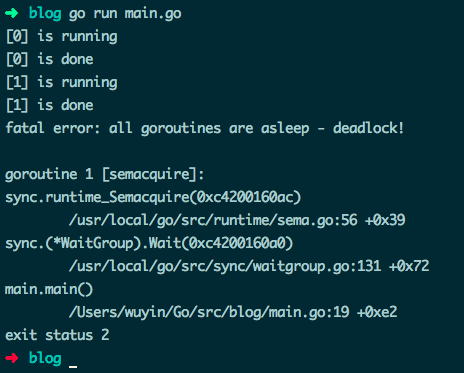fatal error: all goroutines are asleep - deadlock!

// www.topgoer.com go语言中文文档 // 等待所有 goroutine 执行完毕 // 使用传址方式为 WaitGroup 变量传参 // 使用 channel 关闭 goroutine

func main() { var wg sync.WaitGroup done := make(chan struct{}) ch := make(chan interface{})

``````workerCount := 2
for i := 0; i
``````
``````运行效果：

![](http://topgoer.com/static/xiangmu/12.png)

#### 32.向无缓冲的 channel 发送数据，只要 receiver 准备好了就会立刻返回

``````
``````func main() {
ch := make(chan string)

go func() {
for m := range ch {
fmt.Println("Processed:", m)
time.Sleep(1 * time.Second)    // 模拟需要长时间运行的操作
}
}()

ch

``````#### 33.向已关闭的 channel 发送数据会造成 panic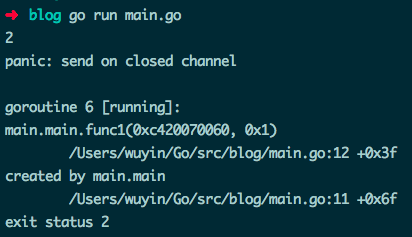func main() { ch := make(chan int) done := make(chan struct{})

``````for i := 0; i
``````
``````运行效果：

![](http://topgoer.com/static/xiangmu/15.png)

#### 34.使用了值为 nil 的 channel

``````

func main() { var ch chan int // 未初始化，值为 nil for i := 0; i

``````runtime 死锁错误：

> fatal error: all goroutines are asleep - deadlock! goroutine 1 \[chan receive (nil chan)\]

``````
``````func main() {
inCh := make(chan int)
outCh := make(chan int)

go func() {
var in

``````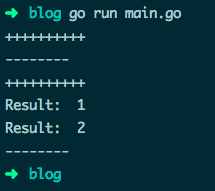#### 35.若函数 receiver 传参是传值方式，则无法修改参数的原有值

``````type data struct {
num   int
key   *string
items map[string]bool
}

func (this *data) pointerFunc() {
this.num = 7
}

func (this data) valueFunc() {
this.num = 8
*this.key = "valueFunc.key"
this.items["valueFunc"] = true
}

func main() {
key := "key1"

d := data{1, &key, make(map[string]bool)}
fmt.Printf("num=%v  key=%v  items=%v\n", d.num, *d.key, d.items)

d.pointerFunc()    // 修改 num 的值为 7
fmt.Printf("num=%v  key=%v  items=%v\n", d.num, *d.key, d.items)

d.valueFunc()    // 修改 key 和 items 的值
fmt.Printf("num=%v  key=%v  items=%v\n", d.num, *d.key, d.items)
}

``````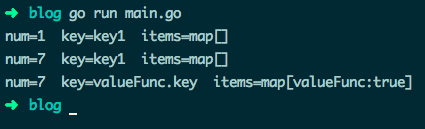### 1.1.2. 中级篇：36-51

#### 36.关闭 HTTP 的响应体

``````// 请求失败造成 panic
func main() {
resp, err := http.Get("https://api.ipify.org?format=json")
defer resp.Body.Close()    // resp 可能为 nil，不能读取 Body
if err != nil {
fmt.Println(err)
return
}

body, err := ioutil.ReadAll(resp.Body)
checkError(err)

fmt.Println(string(body))
}

func checkError(err error) {
if err != nil{
log.Fatalln(err)
}
}

``````

panic: runtime error: invalid memory address or nil pointer dereference

``````// 大多数情况正确的示例
func main() {
resp, err := http.Get("https://api.ipify.org?format=json")
checkError(err)

defer resp.Body.Close()    // 绝大多数情况下的正确关闭方式
body, err := ioutil.ReadAll(resp.Body)
checkError(err)

fmt.Println(string(body))
}

``````

``````Get https://api.ipify.org?format=...: x509: certificate signed by unknown authority

``````

• 可以直接在处理 HTTP 响应错误的代码块中，直接关闭非 nil 的响应体。
• 手动调用 defer 来关闭响应体：
``````// 正确示例
func main() {
resp, err := http.Get("http://www.baidu.com")

// 关闭 resp.Body 的正确姿势
if resp != nil {
defer resp.Body.Close()
}

checkError(err)
defer resp.Body.Close()

body, err := ioutil.ReadAll(resp.Body)
checkError(err)

fmt.Println(string(body))
}

``````

resp.Body.Close() 早先版本的实现是读取响应体的数据之后丢弃，保证了 keep-alive 的 HTTP 连接能重用处理不止一个请求。但 Go 的最新版本将读取并丢弃数据的任务交给了用户，如果你不处理，HTTP 连接可能会直接关闭而非重用，参考在 Go 1.5 版本文档。

``````    _, err = io.Copy(ioutil.Discard, resp.Body) // 手动丢弃读取完毕的数据

``````

``````    json.NewDecoder(resp.Body).Decode(&data)

``````

#### 37.关闭 HTTP 连接

• 直接设置请求变量的 Close 字段值为 true，每次请求结束后就会主动关闭连接。
• 设置 Header 请求头部选项 Connection: close，然后服务器返回的响应头部也会有这个选项，此时 HTTP 标准库会主动断开连接。
``````// 主动关闭连接
func main() {
req, err := http.NewRequest("GET", "http://golang.org", nil)
checkError(err)

req.Close = true

resp, err := http.DefaultClient.Do(req)
if resp != nil {
defer resp.Body.Close()
}
checkError(err)

body, err := ioutil.ReadAll(resp.Body)
checkError(err)

fmt.Println(string(body))
}

``````

``````func main() {
tr := http.Transport{DisableKeepAlives: true}
client := http.Client{Transport: &tr}

resp, err := client.Get("https://golang.google.cn/")
if resp != nil {
defer resp.Body.Close()
}
checkError(err)

fmt.Println(resp.StatusCode)    // 200

body, err := ioutil.ReadAll(resp.Body)
checkError(err)

fmt.Println(len(string(body)))
}

``````

• 若你的程序要向同一服务器发大量请求，使用默认的保持长连接。
• 若你的程序要连接大量的服务器，且每台服务器只请求一两次，那收到请求后直接关闭连接。或增加最大文件打开数 fs.file-max 的值。

#### 38.将 JSON 中的数字解码为 interface 类型

``````func main() {
var data = []byte(`{"status": 200}`)
var result map[string]interface{}

if err := json.Unmarshal(data, &result); err != nil {
log.Fatalln(err)
}

fmt.Printf("%T\n", result["status"])    // float64
var status = result["status"].(int)    // 类型断言错误
fmt.Println("Status value: ", status)
}

``````

panic: interface conversion: interface {} is float64, not int

• 将 int 值转为 float 统一使用
• 将 decode 后需要的 float 值转为 int 使用
``````// 将 decode 的值转为 int 使用
func main() {
var data = []byte(`{"status": 200}`)
var result map[string]interface{}

if err := json.Unmarshal(data, &result); err != nil {
log.Fatalln(err)
}

var status = uint64(result["status"].(float64))
fmt.Println("Status value: ", status)
}

``````
• 使用 Decoder 类型来 decode JSON 数据，明确表示字段的值类型
``````// 指定字段类型
func main() {
var data = []byte(`{"status": 200}`)
var result map[string]interface{}

var decoder = json.NewDecoder(bytes.NewReader(data))
decoder.UseNumber()

if err := decoder.Decode(&result); err != nil {
log.Fatalln(err)
}

var status, _ = result["status"].(json.Number).Int64()
fmt.Println("Status value: ", status)
}

// 你可以使用 string 来存储数值数据，在 decode 时再决定按 int 还是 float 使用
// 将数据转为 decode 为 string
func main() {
var data = []byte({"status": 200})
var result map[string]interface{}
var decoder = json.NewDecoder(bytes.NewReader(data))
decoder.UseNumber()
if err := decoder.Decode(&result); err != nil {
log.Fatalln(err)
}
var status uint64
err := json.Unmarshal([]byte(result["status"].(json.Number).String()), &status);
checkError(err)
fmt.Println("Status value: ", status)
}

``````

``````// struct 中指定字段类型
func main() {
var data = []byte(`{"status": 200}`)
var result struct {
Status uint64 `json:"status"`
}

checkError(err)
fmt.Printf("Result: %+v", result)
}

``````
• 可以使用 struct 将数值类型映射为 json.RawMessage 原生数据类型 适用于如果 JSON 数据不着急 decode 或 JSON 某个字段的值类型不固定等情况：
``````// 状态名称可能是 int 也可能是 string，指定为 json.RawMessage 类型
func main() {
records := [][]byte{
[]byte(`{"status":200, "tag":"one"}`),
[]byte(`{"status":"ok", "tag":"two"}`),
}

for idx, record := range records {
var result struct {
StatusCode uint64
StatusName string
Status     json.RawMessage `json:"status"`
Tag        string          `json:"tag"`
}

checkError(err)

var name string
err = json.Unmarshal(result.Status, &name)
if err == nil {
result.StatusName = name
}

var code uint64
err = json.Unmarshal(result.Status, &code)
if err == nil {
result.StatusCode = code
}

fmt.Printf("[%v] result => %+v\n", idx, result)
}

``````

#### 39.struct、array、slice 和 map 的值比较

``````type data struct {
num     int
fp      float32
complex complex64
str     string
char    rune
yes     bool
events

``````

``````type data struct {
num    int
checks func() bool        // 无法比较
doIt   func() bool        // 无法比较
m      map[string]string    // 无法比较
bytes  []byte            // 无法比较
}

func main() {
v1 := data{}
v2 := data{}

fmt.Println("v1 == v2: ", v1 == v2)
}

``````

invalid operation: v1 == v2 (struct containing func() bool cannot be compared)

Go 提供了一些库函数来比较那些无法使用 == 比较的变量，比如使用 “reflect” 包的 DeepEqual() ：

``````// 比较相等运算符无法比较的元素
func main() {
v1 := data{}
v2 := data{}
fmt.Println("v1 == v2: ", reflect.DeepEqual(v1, v2))    // true

m1 := map[string]string{"one": "a", "two": "b"}
m2 := map[string]string{"two": "b", "one": "a"}
fmt.Println("v1 == v2: ", reflect.DeepEqual(m1, m2))    // true

s1 := []int{1, 2, 3}
s2 := []int{1, 2, 3}
// 注意两个 slice 相等，值和顺序必须一致
fmt.Println("v1 == v2: ", reflect.DeepEqual(s1, s2))    // true
}

``````

``````func main() {
var b1 []byte = nil
b2 := []byte{}
fmt.Println("b1 == b2: ", reflect.DeepEqual(b1, b2))    // false
}

``````

• DeepEqual() 并不总适合于比较 slice
``````func main() {
var str = "one"
var in interface{} = "one"
fmt.Println("str == in: ", reflect.DeepEqual(str, in))    // true

v1 := []string{"one", "two"}
v2 := []string{"two", "one"}
fmt.Println("v1 == v2: ", reflect.DeepEqual(v1, v2))    // false

data := map[string]interface{}{
"code":  200,
"value": []string{"one", "two"},
}
encoded, _ := json.Marshal(data)
var decoded map[string]interface{}
json.Unmarshal(encoded, &decoded)
fmt.Println("data == decoded: ", reflect.DeepEqual(data, decoded))    // false
}

``````

• reflect.DeepEqual() 认为空 slice 与 nil slice 并不相等，但注意 byte.Equal() 会认为二者相等：
``````func main() {
var b1 []byte = nil
b2 := []byte{}

// b1 与 b2 长度相等、有相同的字节序
// nil 与 slice 在字节上是相同的
fmt.Println("b1 == b2: ", bytes.Equal(b1, b2))    // true
}

``````

#### 40.从 panic 中恢复

``````// 错误的 recover 调用示例
func main() {
recover()    // 什么都不会捕捉
panic("not good")    // 发生 panic，主程序退出
recover()    // 不会被执行
println("ok")
}

// 正确的 recover 调用示例
func main() {
defer func() {
fmt.Println("recovered: ", recover())
}()
panic("not good")
}

``````

``````// 错误的调用示例
func main() {
defer func() {
doRecover()
}()
panic("not good")
}

func doRecover() {
fmt.Println("recobered: ", recover())
}

``````

recobered: panic: not good

#### 41.在 range 迭代 slice、array、map 时通过更新引用来更新元素

``````func main() {
data := []int{1, 2, 3}
for _, v := range data {
v *= 10        // data 中原有元素是不会被修改的
}
fmt.Println("data: ", data)    // data:  [1 2 3]
}

``````

``````func main() {
data := []int{1, 2, 3}
for i, v := range data {
data[i] = v * 10
}
fmt.Println("data: ", data)    // data:  [10 20 30]
}

``````

``````func main() {
data := []*struct{ num int }{{1}, {2}, {3},}
for _, v := range data {
v.num *= 10    // 直接使用指针更新
}
fmt.Println(data, data, data)    // &{10} &{20} &{30}
}

``````

#### 42.slice 中隐藏的数据

``````func get() []byte {
raw := make([]byte, 10000)
fmt.Println(len(raw), cap(raw), &raw)    // 10000 10000 0xc420080000
return raw[:3]    // 重新分配容量为 10000 的 slice
}

func main() {
data := get()
fmt.Println(len(data), cap(data), &data)    // 3 10000 0xc420080000
}

``````

``````func get() (res []byte) {
raw := make([]byte, 10000)
fmt.Println(len(raw), cap(raw), &raw)    // 10000 10000 0xc420080000
res = make([]byte, 3)
copy(res, raw[:3])
return
}

func main() {
data := get()
fmt.Println(len(data), cap(data), &data)    // 3 3 0xc4200160b8
}

``````

#### 43.Slice 中数据的误用

``````// 错误使用 slice 的拼接示例
func main() {
path := []byte("AAAA/BBBBBBBBB")
sepIndex := bytes.IndexByte(path, '/') // 4
println(sepIndex)

dir1 := path[:sepIndex]
dir2 := path[sepIndex+1:]
println("dir1: ", string(dir1))        // AAAA
println("dir2: ", string(dir2))        // BBBBBBBBB

dir1 = append(dir1, "suffix"...)
println("current path: ", string(path))    // AAAAsuffixBBBB

path = bytes.Join([][]byte{dir1, dir2}, []byte{'/'})
println("dir1: ", string(dir1))        // AAAAsuffix
println("dir2: ", string(dir2))        // uffixBBBB

println("new path: ", string(path))    // AAAAsuffix/uffixBBBB    // 错误结果
}

``````

• 重新分配新的 slice 并拷贝你需要的数据
• 使用完整的 slice 表达式：input[low:high:max]，容量便调整为 max - low
``````// 使用 full slice expression
func main() {

path := []byte("AAAA/BBBBBBBBB")
sepIndex := bytes.IndexByte(path, '/') // 4
dir1 := path[:sepIndex:sepIndex]        // 此时 cap(dir1) 指定为4， 而不是先前的 16
dir2 := path[sepIndex+1:]
dir1 = append(dir1, "suffix"...)

path = bytes.Join([][]byte{dir1, dir2}, []byte{'/'})
println("dir1: ", string(dir1))        // AAAAsuffix
println("dir2: ", string(dir2))        // BBBBBBBBB
println("new path: ", string(path))    // AAAAsuffix/BBBBBBBBB
}

``````

#### 44.旧 slice

``````// 超过容量将重新分配数组来拷贝值、重新存储
func main() {
s1 := []int{1, 2, 3}
fmt.Println(len(s1), cap(s1), s1)    // 3 3 [1 2 3 ]

s2 := s1[1:]
fmt.Println(len(s2), cap(s2), s2)    // 2 2 [2 3]

for i := range s2 {
s2[i] += 20
}
// 此时的 s1 与 s2 是指向同一个底层数组的
fmt.Println(s1)        // [1 22 23]
fmt.Println(s2)        // [22 23]

s2 = append(s2, 4)    // 向容量为 2 的 s2 中再追加元素，此时将分配新数组来存

for i := range s2 {
s2[i] += 10
}
fmt.Println(s1)        // [1 22 23]    // 此时的 s1 不再更新，为旧数据
fmt.Println(s2)        // [32 33 14]
}

``````

#### 45.类型声明与方法

``````// 定义 Mutex 的自定义类型
type myMutex sync.Mutex

func main() {
var mtx myMutex
mtx.Lock()
mtx.UnLock()
}

``````

mtx.Lock undefined (type myMutex has no field or method Lock)…

``````// 类型以字段形式直接嵌入
type myLocker struct {
sync.Mutex
}

func main() {
var locker myLocker
locker.Lock()
locker.Unlock()
}

``````

interface 类型声明也保留它的方法集：

``````type myLocker sync.Locker

func main() {
var locker myLocker
locker.Lock()
locker.Unlock()
}

``````

#### 46.跳出 for-switch 和 for-select 代码块

``````// break 配合 label 跳出指定代码块
func main() {
loop:
for {
switch {
case true:
fmt.Println("breaking out...")
//break    // 死循环，一直打印 breaking out...
break loop
}
}
fmt.Println("out...")
}

``````

goto 虽然也能跳转到指定位置，但依旧会再次进入 for-switch，死循环。

#### 47.for 语句中的迭代变量与闭包函数

for 语句中的迭代变量在每次迭代中都会重用，即 for 中创建的闭包函数接收到的参数始终是同一个变量，在 goroutine 开始执行时都会得到同一个迭代值：

``````func main() {
data := []string{"one", "two", "three"}

for _, v := range data {
go func() {
fmt.Println(v)
}()
}

time.Sleep(3 * time.Second)
// 输出 three three three
}

``````

``````func main() {
data := []string{"one", "two", "three"}

for _, v := range data {
vCopy := v
go func() {
fmt.Println(vCopy)
}()
}

time.Sleep(3 * time.Second)
// 输出 one two three
}

``````

``````func main() {
data := []string{"one", "two", "three"}

for _, v := range data {
go func(in string) {
fmt.Println(in)
}(v)
}

time.Sleep(3 * time.Second)
// 输出 one two three
}

``````

``````type field struct {
name string
}

func (p *field) print() {
fmt.Println(p.name)
}

// 错误示例
func main() {
data := []field{{"one"}, {"two"}, {"three"}}
for _, v := range data {
go v.print()
}
time.Sleep(3 * time.Second)
// 输出 three three three
}

// 正确示例
func main() {
data := []field{{"one"}, {"two"}, {"three"}}
for _, v := range data {
v := v
go v.print()
}
time.Sleep(3 * time.Second)
// 输出 one two three
}

// 正确示例
func main() {
data := []*field{{"one"}, {"two"}, {"three"}}
for _, v := range data {    // 此时迭代值 v 是三个元素值的地址，每次 v 指向的值不同
go v.print()
}
time.Sleep(3 * time.Second)
// 输出 one two three
}

``````

#### 48.defer 函数的参数值

``````// 在 defer 函数中参数会提前求值
func main() {
var i = 1
defer fmt.Println("result: ", func() int { return i * 2 }())
i++
}

``````

result: 2

#### 49.defer 函数的执行时机

``````// www.topgoer.com go语言中文文档
// 命令行参数指定目录名
// 遍历读取目录下的文件
func main() {

if len(os.Args) != 2 {
os.Exit(1)
}

dir := os.Args
start, err := os.Stat(dir)
if err != nil || !start.IsDir() {
os.Exit(2)
}

var targets []string
filepath.Walk(dir, func(fPath string, fInfo os.FileInfo, err error) error {
if err != nil {
return err
}

if !fInfo.Mode().IsRegular() {
return nil
}

targets = append(targets, fPath)
return nil
})

for _, target := range targets {
f, err := os.Open(target)
if err != nil {
fmt.Println("bad target:", target, "error:", err)    //error:too many open files
break
}
defer f.Close()    // 在每次 for 语句块结束时，不会关闭文件资源

// 使用 f 资源
}
}

``````

``````#!/bin/bash
for n in {1..10000}; do
echo content > "file\${n}.txt"
done

``````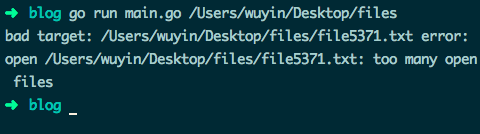``````// 目录遍历正常
func main() {
// ...

for _, target := range targets {
func() {
f, err := os.Open(target)
if err != nil {
fmt.Println("bad target:", target, "error:", err)
return    // 在匿名函数内使用 return 代替 break 即可
}
defer f.Close()    // 匿名函数执行结束，调用关闭文件资源

// 使用 f 资源
}()
}
}

``````

#### 50.失败的类型断言

``````// 错误示例
func main() {
var data interface{} = "great"

// data 混用
if data, ok := data.(int); ok {
fmt.Println("[is an int], data: ", data)
} else {
fmt.Println("[not an int], data: ", data)    // [isn't a int], data:  0
}
}

// 正确示例
func main() {
var data interface{} = "great"

if res, ok := data.(int); ok {
fmt.Println("[is an int], data: ", res)
} else {
fmt.Println("[not an int], data: ", data)    // [not an int], data:  great
}
}

``````

#### 51.阻塞的 gorutinue 与资源泄露

``````func First(query string, replicas []Search) Result {
c := make(chan Result)
replicaSearch := func(i int) { c

``````

• 使用带缓冲的 channel，确保能接收全部 goroutine 的返回结果：
``````func First(query string, replicas ...Search) Result {
c := make(chan Result,len(replicas))
searchReplica := func(i int) { c

``````
• 使用 select 语句，配合能保存一个缓冲值的 channel default 语句： default 的缓冲 channel 保证了即使结果 channel 收不到数据，也不会阻塞 goroutine
``````func First(query string, replicas ...Search) Result {
c := make(chan Result,1)
searchReplica := func(i int) {
select {
case c

``````
• 使用特殊的废弃（cancellation） channel 来中断剩余 goroutine 的执行：
``````func First(query string, replicas ...Search) Result {
c := make(chan Result)
done := make(chan struct{})
defer close(done)
searchReplica := func(i int) {
select {
case c

``````

Rob Pike 为了简化演示，没有提及演讲代码中存在的这些问题。不过对于新手来说，可能会不加思考直接使用。

### 1.1.3. 高级篇：52-58

``````type data struct {
name string
}

type printer interface {
print()
}

func (p *data) print() {
fmt.Println("name: ", p.name)
}

func main() {
d1 := data{"one"}
d1.print()    // d1 变量可寻址，可直接调用指针 receiver 的方法

var in printer = data{"two"}
in.print()    // 类型不匹配

m := map[string]data{
"x": data{"three"},
}
m["x"].print()    // m["x"] 是不可寻址的    // 变动频繁
}

``````

cannot use data literal (type data) as type printer in assignment: data does not implement printer (print method has pointer receiver)

cannot call pointer method on m[“x”] cannot take the address of m[“x”]

#### 53.更新 map 字段的值

``````// 无法直接更新 struct 的字段值
type data struct {
name string
}

func main() {
m := map[string]data{
"x": {"Tom"},
}
m["x"].name = "Jerry"
}

``````

cannot assign to struct field m[“x”].name in map

``````type data struct {
name string
}

func main() {
s := []data{{"Tom"}}
s.name = "Jerry"
fmt.Println(s)    // [{Jerry}]
}

``````

• 使用局部变量
``````// 提取整个 struct 到局部变量中，修改字段值后再整个赋值
type data struct {
name string
}

func main() {
m := map[string]data{
"x": {"Tom"},
}
r := m["x"]
r.name = "Jerry"
m["x"] = r
fmt.Println(m)    // map[x:{Jerry}]
}

``````
• 使用指向元素的 map 指针
``````func main() {
m := map[string]*data{
"x": {"Tom"},
}

m["x"].name = "Jerry"    // 直接修改 m["x"] 中的字段
fmt.Println(m["x"])    // &{Jerry}
}

``````

``````func main() {
m := map[string]*data{
"x": {"Tom"},
}
m["z"].name = "what???"
fmt.Println(m["x"])
}

``````

panic: runtime error: invalid memory address or nil pointer dereference

#### 54.nil interface 和 nil interface 值

``````func main() {
var data *byte
var in interface{}

fmt.Println(data, data == nil)    //  true
fmt.Println(in, in == nil)    //  true

in = data
fmt.Println(in, in == nil)    //  false    // data 值为 nil，但 in 值不为 nil
}

``````

``````// 错误示例
func main() {
doIt := func(arg int) interface{} {
var result *struct{} = nil
if arg > 0 {
result = &struct{}{}
}
return result
}

if res := doIt(-1); res != nil {
fmt.Println("Good result: ", res)    // Good result:
fmt.Printf("%T\n", res)            // *struct {}    // res 不是 nil，它的值为 nil
fmt.Printf("%v\n", res)            //
}
}

// 正确示例
func main() {
doIt := func(arg int) interface{} {
var result *struct{} = nil
if arg > 0 {
result = &struct{}{}
} else {
return nil    // 明确指明返回 nil
}
return result
}

if res := doIt(-1); res != nil {
fmt.Println("Good result: ", res)
} else {
fmt.Println("Bad result: ", res)    // Bad result:
}
}

``````

#### 55.堆栈变量

Go 编译器会根据变量的大小及其 “escape analysis” 的结果来决定变量的存储位置，故能准确返回本地变量的地址，这在 C/C++ 中是不行的。#### 56.GOMAXPROCS、Concurrency（并发）and Parallelism（并行）

Go 1.4 及以下版本，程序只会使用 1 个执行上下文 / OS 线程，即任何时间都最多只有 1 个 goroutine 在执行。

Go 1.5 版本将可执行上下文的数量设置为 runtime.NumCPU() 返回的逻辑 CPU 核心数，这个数与系统实际总的 CPU 逻辑核心数是否一致，取决于你的 CPU 分配给程序的核心数，可以使用 GOMAXPROCS 环境变量或者动态的使用 runtime.GOMAXPROCS() 来调整。

GOMAXPROCS 的值是可以超过 CPU 的实际数量的，在 1.5 中最大为 256

``````func main() {
fmt.Println(runtime.GOMAXPROCS(-1))    // 4
fmt.Println(runtime.NumCPU())    // 4
runtime.GOMAXPROCS(20)
fmt.Println(runtime.GOMAXPROCS(-1))    // 20
runtime.GOMAXPROCS(300)
fmt.Println(runtime.GOMAXPROCS(-1))    // Go 1.9.2 // 300
}

``````

#### 57.读写操作的重新排序

Go 可能会重排一些操作的执行顺序，可以保证在一个 goroutine 中操作是顺序执行的，但不保证多 goroutine 的执行顺序：

``````var _ = runtime.GOMAXPROCS(3)

var a, b int

func u1() {
a = 1
b = 2
}

func u2() {
a = 3
b = 4
}

func p() {
println(a)
println(b)
}

func main() {
go u1()    // 多个 goroutine 的执行顺序不定
go u2()
go p()
time.Sleep(1 * time.Second)
}

``````#### 58.优先调度

``````func main() {
done := false

go func() {
done = true
}()

for !done {
}

println("done !")
}

``````

for 的循环体不必为空，但如果代码不会触发调度器执行，将出现问题。

``````func main() {
done := false

go func() {
done = true
}()

for !done {
println("not done !")    // 并不内联执行
}

println("done !")
}

``````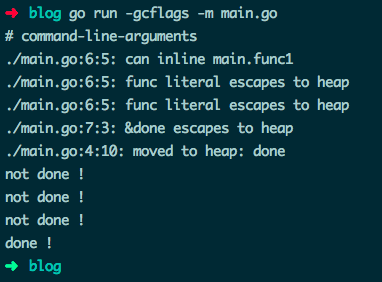``````func main() {
done := false

go func() {
done = true
}()

for !done {
runtime.Gosched()
}

println("done !")
}

``````markdown 9ong@TsingChan